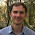## Thursday, January 14, 2016

### Refactoring in Swift - explaining variable

Swift theory

In case you are not a Swift programmer, CGRect is a structure that defines a rectangle by setting its origin and size.

For example, a CGRect of value (10, 20, 100, 150) represents a rectangle with origin at x = 10 and y = 20 and with a size of width = 100 and height = 150.

Refactoring

Now, let's build a game. Or at least a very small part of the game.

let myShipFrame = CGRect(x: 0, y: 0, width: 100, height: 100)
let enemyShipFrame = CGRect(x: 50, y: 50, width: 100, height: 100)

if abs((myShipFrame.origin.x + myShipFrame.width/2.0) - (enemyShipFrame.origin.x + enemyShipFrame.width/2.0)) < (myShipFrame.width/2.0 + enemyShipFrame.width/2.0) {
gameFinished = true
}

Huh?!?

Exactly!

We understand that we have two ships, each of them defined as a rectangle (CGRect) and that we decide somehow if the game is finished.

How that is done is not that clear.

In this situation, to make the code more obvious, we can introduce a variable that has only one purpose: to explain the code.

Let's change the above code as follows:

let shipCollided = abs((myShipFrame.origin.x + myShipFrame.width/2.0) - (enemyShipFrame.origin.x + enemyShipFrame.width/2.0)) < (myShipFrame.width/2.0 + enemyShipFrame.width/2.0)

if shipCollided {
gameFinished = true
}

Oh, now I get it: the long formula is for deciding if the two ships collided. This helps a lot.

But we can go even further. We understand what is the formula for but we are not really understanding how it is calculating this fact. So we can add few more explaining variables:

let myShipCenter = myShipFrame.origin.x + myShipFrame.width/2.0
let enemyShipCenter = enemyShipFrame.origin.x + enemyShipFrame.width/2.0
let minimumDistanceForCollision = myShipFrame.width/2.0 + enemyShipFrame.width/2.0
let shipCollided = abs(myShipCenter - enemyShipCenter) < minimumDistanceForCollision

if shipCollided {
gameFinished = true
}

Without looking at the calculations we understand that the two ships collide if the distance between the centres of the ships (that is abs(myShipCenter - enemyShipCenter)) is smaller than the minimum distance for collision (minimumDistanceForCollision).

Now the code is way more obvious than the first version.

And, of course, we realize the code has a bug as it is considering only X axis and the same check needs to be done on Y axis. But that is your homework :-)

1.2.